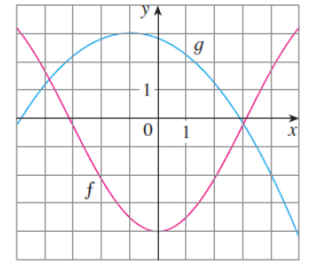Chapter 1.3, Problem 54E### Single Variable Calculus: Early Tr...

8th Edition
James Stewart
ISBN: 9781305270343

#### Solutions

Chapter
Section### Single Variable Calculus: Early Tr...

8th Edition
James Stewart
ISBN: 9781305270343
Textbook Problem

# Use the given graphs of f and g to estimate the value of f(g(x)) for x = − 5, − 4, − 3,. . . , 5. Use these estimates to sketch a rough graph of f ◦ g.To determine

To estimate: The value of f(g(x)) for x=5,4,3,2,1,0,1,2,3,4,5 from the graphs of f and g; using these values sketch the graph of fg.

Explanation

First find the value of g(x).

Note that at x=5, the graph g takes the value of −0.2.

Similarly, from the graph g, the values of g(x) is listed below.

 x g(x) −5 −0.2 −4 1.2 −3 2.2 −2 2.8 −1 3 0 2.8 1 2.2 2 1.2 3 −0.2 4 −1.9 5 −4.1

Table 1

Note that at x=0.2, the graph f takes the value of −4.

Similarly, from the graph f, the values of f(g(x)) is listed below.

 x g(x) f(g(x)) −5 −0

### Still sussing out bartleby?

Check out a sample textbook solution.

See a sample solution

#### The Solution to Your Study Problems

Bartleby provides explanations to thousands of textbook problems written by our experts, many with advanced degrees!

Get Started